RRB ALP & Technician Mock Test (English) - 4

# RRB ALP & Technician Mock Test (English) - 4

Test Description

## 75 Questions MCQ Test RRB ALP & Technician Exam (Group C ) - Mock Tests | RRB ALP & Technician Mock Test (English) - 4

RRB ALP & Technician Mock Test (English) - 4 for Railways 2023 is part of RRB ALP & Technician Exam (Group C ) - Mock Tests preparation. The RRB ALP & Technician Mock Test (English) - 4 questions and answers have been prepared according to the Railways exam syllabus.The RRB ALP & Technician Mock Test (English) - 4 MCQs are made for Railways 2023 Exam. Find important definitions, questions, notes, meanings, examples, exercises, MCQs and online tests for RRB ALP & Technician Mock Test (English) - 4 below.
Solutions of RRB ALP & Technician Mock Test (English) - 4 questions in English are available as part of our RRB ALP & Technician Exam (Group C ) - Mock Tests for Railways & RRB ALP & Technician Mock Test (English) - 4 solutions in Hindi for RRB ALP & Technician Exam (Group C ) - Mock Tests course. Download more important topics, notes, lectures and mock test series for Railways Exam by signing up for free. Attempt RRB ALP & Technician Mock Test (English) - 4 | 75 questions in 60 minutes | Mock test for Railways preparation | Free important questions MCQ to study RRB ALP & Technician Exam (Group C ) - Mock Tests for Railways Exam | Download free PDF with solutions
 1 Crore+ students have signed up on EduRev. Have you?
RRB ALP & Technician Mock Test (English) - 4 - Question 1

### Which of the following correctly represents the molecular formula of washing soda?

RRB ALP & Technician Mock Test (English) - 4 - Question 2

### The ratio between the present ages of A and B is 4:5. If the difference between their present ages is 8 yrs, what is the sum of their present ages?

Detailed Solution for RRB ALP & Technician Mock Test (English) - 4 - Question 2

The ratio between the present ages of A and B is 4:5.
A/B = 4/5 ⇒ 5A = 4B ⇒ 5A - 4B = 0 .....(1)
If the difference between their present ages is 8 yrs
A - B = 8 .....(2)
multiplying 5 both sides in equation(2)
⇒ 5(A - B) = 40
⇒ 5A - 5B = 40
Subtracting equation (1) from equation (2) we get
B = 40
5A = 4B
⇒ 5A = 4 x 40 = 160
⇒ A = 160/5 = 32
Sum of present ages = 40 + 32 = 72

RRB ALP & Technician Mock Test (English) - 4 - Question 3

### Find the same relationship among the four alternative numbers given under it. 8 : 81 : : 64 : ?

RRB ALP & Technician Mock Test (English) - 4 - Question 4
Animals that are viviparous and nourish their young ones with milk are :
RRB ALP & Technician Mock Test (English) - 4 - Question 5

The minute hand of clock is √21 cm long. The area described by a minute hand on the face of the clock between 7 am and 7.05 am is

Detailed Solution for RRB ALP & Technician Mock Test (English) - 4 - Question 5

Given that length of minute hand of clock is √21 cm
Angle described by the minute hand in 60 minutes = 360o
Angle described by the minute hand in 5 minutes = 360/60 x 5 = 30o

We know that Area of sector = θ/360 x πr2
= 30/360 x 22/7 x √21 x √21
= 11/2 = 5.5 cm2

RRB ALP & Technician Mock Test (English) - 4 - Question 6
Find the correct combination
Renuka's mother is the only daughter of Mini's husband related to Renuka ?
RRB ALP & Technician Mock Test (English) - 4 - Question 7
An unsaturated hydrocarbon :
RRB ALP & Technician Mock Test (English) - 4 - Question 8
Today is 1st April. The day of the week is Wednesday. This is a leap year. The day of the week on this day after 3 years will be
Detailed Solution for RRB ALP & Technician Mock Test (English) - 4 - Question 8 This year is a leap year so next three years will be ordinary years. Hence, 3 odd days.
RRB ALP & Technician Mock Test (English) - 4 - Question 9
'Palade particles 'is another name of which of the following cell organelles?
RRB ALP & Technician Mock Test (English) - 4 - Question 10
Which of the following is not a combination reaction?
RRB ALP & Technician Mock Test (English) - 4 - Question 11
Choose the word which is least like the other words in the group .
RRB ALP & Technician Mock Test (English) - 4 - Question 12
If REASON is coded as 5 and BELIEVED as 7, what is the code number for GOVERNMENT ?
RRB ALP & Technician Mock Test (English) - 4 - Question 13
Choose the word which is least like the other words in the group .
RRB ALP & Technician Mock Test (English) - 4 - Question 14
If in a code language, COULD is written as BNTKC and MARGIN is written as LZQFHM, how will MOULDING be written in that code ?
RRB ALP & Technician Mock Test (English) - 4 - Question 15
Complete the analogous pair.
Voter : 18 years :: Lok Sabha Member : ?
RRB ALP & Technician Mock Test (English) - 4 - Question 16

A sum of moeny doubles itself in 10 years at simple interest. What is the rate of interest ?

Detailed Solution for RRB ALP & Technician Mock Test (English) - 4 - Question 16

Let sum of money be x
Then SI = x
⇒ SI = P x R x T/100
⇒ R = SI x 100/P x 10 = 10%

RRB ALP & Technician Mock Test (English) - 4 - Question 17
The ‘AC-21’ law passed by the US Congress governs
RRB ALP & Technician Mock Test (English) - 4 - Question 18
The Dodd-Frank Act passed by the US Congress in 2010 has a section that declares certain minerals as ‘conflict minerals’. Which one of the following minerals is NOT one among them?
RRB ALP & Technician Mock Test (English) - 4 - Question 19
The argument put forth by the tobacco industry against the use of 85 per cent packaging space on tobacco products for pictorial warnings is that
RRB ALP & Technician Mock Test (English) - 4 - Question 20
Noted educational administrator Satish Chandra passed away recently. He wrote which subject’s NCERT’s textbooks in 1970s?
RRB ALP & Technician Mock Test (English) - 4 - Question 21
Where did the 5th India-Sri Lanka joint training exercise “Mitra Shakti 2017” conducted in October 2017?
RRB ALP & Technician Mock Test (English) - 4 - Question 22
What was the theme of the 2017 International Migrants Day?
RRB ALP & Technician Mock Test (English) - 4 - Question 23
Going towards north a Police-Inspector takes a right turn and goes 5 kms straight. He then turns right again and goes 8 km straight and finally he takes a left turn to reach his office. In which direction is his office from the starting point?
Detailed Solution for RRB ALP & Technician Mock Test (English) - 4 - Question 23 The route of the Police Inspector is as given below:
RRB ALP & Technician Mock Test (English) - 4 - Question 24
According to the latest census conducted by the Reserve Bank of India (RBI), which country emerged as the largest source of FDI in India in 2016-17?
RRB ALP & Technician Mock Test (English) - 4 - Question 25
The device used for measuring potential difference is known as
RRB ALP & Technician Mock Test (English) - 4 - Question 26
What does 'come' represent in a code language ?
I. 'pit na tac' means 'come and go' in that code language.
II. 'ja ta da' means 'you are good' in that code language.
III. 'na da rac' means 'you can come' in that code language.
The question given above has a problem and three statements giving certain information. you have to decide if the information given in the statements is sufficient for answering the problem. Indicate your answer as
Detailed Solution for RRB ALP & Technician Mock Test (English) - 4 - Question 26
RRB ALP & Technician Mock Test (English) - 4 - Question 27

If 71 + x + 71 − x = 50, then x =

Detailed Solution for RRB ALP & Technician Mock Test (English) - 4 - Question 27

7(7x) + 7/7x  = 50

Let 7x  = y

RRB ALP & Technician Mock Test (English) - 4 - Question 28
If x = r sin θ cos φ, y = r sin θ sin φ, z = r cos θ then x2 + y2 + z2 =
RRB ALP & Technician Mock Test (English) - 4 - Question 29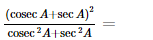RRB ALP & Technician Mock Test (English) - 4 - Question 30
There are 36 students in a class. If two-third of them are boys and three-fourth of the boys are under 6 feet tall how many boys in the class are under six feet tall?
RRB ALP & Technician Mock Test (English) - 4 - Question 31
Staple food crops, such as potatoes, are low in
RRB ALP & Technician Mock Test (English) - 4 - Question 32
When an object undergoes accelerationits speed always increases
RRB ALP & Technician Mock Test (English) - 4 - Question 33

AB∥CD and P is a point between the lines, the measure of the angles ∠ABP+∠BPD+∠CDP is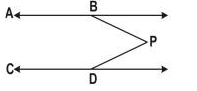RRB ALP & Technician Mock Test (English) - 4 - Question 34

If the ratio of the areas of two similar triangles is 48:32, then the ratio of the lengths of their corresponding sides is

RRB ALP & Technician Mock Test (English) - 4 - Question 35
What is the acceleration produced in a freely falling body of mass 10 kg? (Neglect air resistance)
RRB ALP & Technician Mock Test (English) - 4 - Question 36

The HCF and LCM of two numbers are 12 and 72 respectively. If the sum of the two numbers is 60,then one of the two numbers will be

Detailed Solution for RRB ALP & Technician Mock Test (English) - 4 - Question 36

H.C.F = 12, L.C.M = 72
Let one number be = x, then other number = 60 - x
Now, x x (60 - x) = 12 x 72
or 60x - x2 = 864
or x2 - 60x + 864 = 0
or (x - 36) (x - 24) = 0
or x = 36 , 24

RRB ALP & Technician Mock Test (English) - 4 - Question 37
Who is known as the father of genetics ?
RRB ALP & Technician Mock Test (English) - 4 - Question 38
In line with United States, which among the given countries has also filed a notice to withdraw its membership from the United Nations Educational, Scientific and Cultural Organization (UNESCO)?
RRB ALP & Technician Mock Test (English) - 4 - Question 39
Find out the missing letter and mark the correct option as answer .
A C E __ I K
RRB ALP & Technician Mock Test (English) - 4 - Question 40
Zygote is formed after:
RRB ALP & Technician Mock Test (English) - 4 - Question 41
Consider the Venn diagram given below :

The numbers in the Venn diagram are persons reading the newspapers indicated. The diagram is drawn after surveying 50 persons. In a population of 10,000 how many can be expected to read at least two newspaper ?
RRB ALP & Technician Mock Test (English) - 4 - Question 42
A well cut diamond appears bright because :
RRB ALP & Technician Mock Test (English) - 4 - Question 43

In questions below, equations have become wrong due to wrong orders of signs. Choose the correct order of signs from the alternative given.

56×6÷3=29-2

RRB ALP & Technician Mock Test (English) - 4 - Question 44
The best method to separate the components of an ink is
RRB ALP & Technician Mock Test (English) - 4 - Question 45
The process of converting hydrated alumina into anhydrous alumina is called :
RRB ALP & Technician Mock Test (English) - 4 - Question 46
Negative acceleration means an object is moving with ________ .
RRB ALP & Technician Mock Test (English) - 4 - Question 47
How much amount of financial assistance has been sanctioned by the Union Government to eight Naxal affected districts of Chhattisgarh for three years under its Special Central Assistance Scheme?
RRB ALP & Technician Mock Test (English) - 4 - Question 48
Which is the Number that comes next in the series:
36, 34, 30, 28, 24 .?
Detailed Solution for RRB ALP & Technician Mock Test (English) - 4 - Question 48 This is an alternating number subtraction series. First, 2 is subtracted, then 4, then 2, and so on.
RRB ALP & Technician Mock Test (English) - 4 - Question 49
A number when divided successively by 4 and 5 leaves remainder 1 and 4 respectively. When it is successively divided by 5 and 4 the respective remainders will be
Detailed Solution for RRB ALP & Technician Mock Test (English) - 4 - Question 49 When dividing a positive integer n by another positive integer D (divider), we obtain a quotient Q, which is a non-negative integer and a remainder R, which is an integer such that 0 ≤ R < D. We can write n = DQ + R.
When dividing our number n by 4 we obtain a remainder of 1, so, if the quotient is some integer Q, we can write n = 4Q + 1.
successively divide means quotient Q is divide by 5.
Now, dividing Q by 5, we obtain another quotient say q and remainder 4, thus we can write Q = 5q + 4.
It follows that n = 4(5q + 4) + 1 = 20q + 17.
Since n = 20q + 17 = 5(4q + 3) + 2, it means that when dividing n by 5 first, we get a quotient 4q + 3 and remainder 2.
Then dividing 4q + 3 by 4 we obviously obtain a remainder of 3.
Hence remainder is 2,3 by dividing the no by 5,4.
RRB ALP & Technician Mock Test (English) - 4 - Question 50
When 24 is added to a number, it increases to its 60%. What is 75% of that number?
RRB ALP & Technician Mock Test (English) - 4 - Question 51
Charu Rohatgi passed away due to health issues. She was a veteran__________
RRB ALP & Technician Mock Test (English) - 4 - Question 52
A tap fills a tank in 12 hrs and the other empties it in 24 hrs. If both are opened simultaneously, then the tank will be filled in
RRB ALP & Technician Mock Test (English) - 4 - Question 53
A person sells 320 mangoes at the cost price of 400 mangoes. What is the profit percent?
RRB ALP & Technician Mock Test (English) - 4 - Question 54
If (5a+3b)/(2a-3b)=23/5, then the value of a:b is
RRB ALP & Technician Mock Test (English) - 4 - Question 55
Grafting is most successful in
RRB ALP & Technician Mock Test (English) - 4 - Question 56

Mr. kalia invested a total amount of Rs. 16500 for two years in two schemes A and B with rate of simple interest 10% per annum and 12% per annum, respectively. If the total amount of interest earned was Rs.3620, what was amount invested in scheme A?

Detailed Solution for RRB ALP & Technician Mock Test (English) - 4 - Question 56

% interest on total amount per annum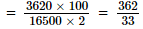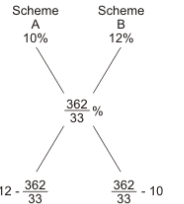Hence, ratio of amount invested in schemes
A and B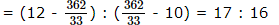Hence, amount invested in A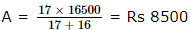RRB ALP & Technician Mock Test (English) - 4 - Question 57

100+50x2=?

RRB ALP & Technician Mock Test (English) - 4 - Question 58

253 ÷ 11 of 23=?

RRB ALP & Technician Mock Test (English) - 4 - Question 59
The recently launched book titled ‘Imperfect’ is the autobiography of which renowned cricketer?
RRB ALP & Technician Mock Test (English) - 4 - Question 60

What should come in the place of the (?) in the following equation? 28/? = ?/112

RRB ALP & Technician Mock Test (English) - 4 - Question 61
Below is given statement followed by two arguments numbered I and II. You have to consider the statement and the following arguments and decide which of the arguments is strong in the statement.
Statement :
Should government establishd higher level Institutes of Technology (IITs) be privatised?
Arguments :
I. Yes, privatisation will make these institutes financially healthy, competitive and quality conscious.
II. Yes, privatisation is the key of the new era-can we survive without it.
RRB ALP & Technician Mock Test (English) - 4 - Question 62
In each question a statement followed by two assumptions numbered I and II is given. Consider the statement and decide which of the given assumption is implicit.
Statement : The two countries have signed a fragile pact, but the vital sovereignity issue remains unresolved.
Assumptions : I. The two countries cannot have permanent peace pact.
II. The two countries may become hostile again after a short spell of time.
RRB ALP & Technician Mock Test (English) - 4 - Question 63
Below is given statement followed by four conclusions numbered I, II, III and IV. You have to consider the statement and the following conclusions and decide which of the conclusions is follows in the statement :
Statements : a. Some buses are rivers.
b. All rivers are mountains.
Conclusions : I. Some mountains are buses.
RRB ALP & Technician Mock Test (English) - 4 - Question 64
Which one of the following average is most affected of extreme observations?
RRB ALP & Technician Mock Test (English) - 4 - Question 65

If v is the variance and σ is the standard deviation, then

RRB ALP & Technician Mock Test (English) - 4 - Question 66
Two rockets approach each other, one at 42000 mph and the other at 18000 mph. They start 3256 miles apart. How far are they apart 1 minute before impact?
RRB ALP & Technician Mock Test (English) - 4 - Question 67
Below are the statements followed by four conclusions numbered I,II,III and IV. You have to consider the statements and the following conclusions and decide which of the conclusion(s) follows the statement(s).
Statements :
a. Some lice are slates.
b. All slates are apples.
c. No apple is car.
Conclusions :
I. Some cars are slates.
II. Some lice are cars.
III. Some apples are lice.
IV. No car is lice.
RRB ALP & Technician Mock Test (English) - 4 - Question 68
Suman can do a work in 3 days. Sumati can do the same work in 2 days. Both of them finish the work together and get Rs 150. What is the share of Suman?
RRB ALP & Technician Mock Test (English) - 4 - Question 69
Seeds develop from which part of a plant ?
RRB ALP & Technician Mock Test (English) - 4 - Question 70
The length of longest pole that can be kept in a room 12 m long, 9 m broad and 8 m high, is .....
RRB ALP & Technician Mock Test (English) - 4 - Question 71
Velocity of sound waves in vacuum is:
RRB ALP & Technician Mock Test (English) - 4 - Question 72
Choose one word which can be formed from the letters of the given word.
MEASUREMENT
RRB ALP & Technician Mock Test (English) - 4 - Question 73
A 1 kg mass has a kinetic energy of 1 joule when its speed is
RRB ALP & Technician Mock Test (English) - 4 - Question 74

There are six teachers A, B, C, D, E and F in a school. Each of the teachers teaches two subjects, one compulsory subject and the other optional subject. D's optional subject is History while three others have it as compulsory subject. E and F have Physics as one of their subjects. F's compulsory subject is Mathematics which is an optional subject of both C and E. History and English are A's subjects but in terms of compulsory and optional subjects, they are reverse of those of D's. Chemistry is an optional subject of any one of them. There is only one female teacher in the school who has English as her compulsory subject.

Q. What is C's compulsory subject?

Detailed Solution for RRB ALP & Technician Mock Test (English) - 4 - Question 74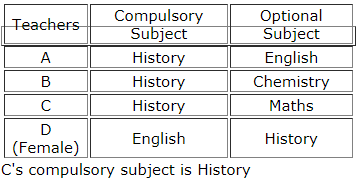RRB ALP & Technician Mock Test (English) - 4 - Question 75

There are six teachers A, B, C, D, E and F in a school. Each of the teachers teaches two subjects, one compulsory subject and the other optional subject. D's optional subject is History while three others have it as compulsory subject. E and F have Physics as one of their subjects. F's compulsory subject is Mathematics which is an optional subject of both C and E. History and English are A's subjects but in terms of compulsory and optional subjects, they are reverse of those of D's. Chemistry is an optional subject of any one of them. There is only one female teacher in the school who has English as her compulsory subject.

Q. Who is a female member in the group?

Detailed Solution for RRB ALP & Technician Mock Test (English) - 4 - Question 75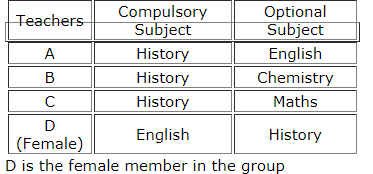## RRB ALP & Technician Exam (Group C ) - Mock Tests

1 docs|65 tests
Information about RRB ALP & Technician Mock Test (English) - 4 Page
In this test you can find the Exam questions for RRB ALP & Technician Mock Test (English) - 4 solved & explained in the simplest way possible. Besides giving Questions and answers for RRB ALP & Technician Mock Test (English) - 4, EduRev gives you an ample number of Online tests for practice

## RRB ALP & Technician Exam (Group C ) - Mock Tests

1 docs|65 tests(Scan QR code)